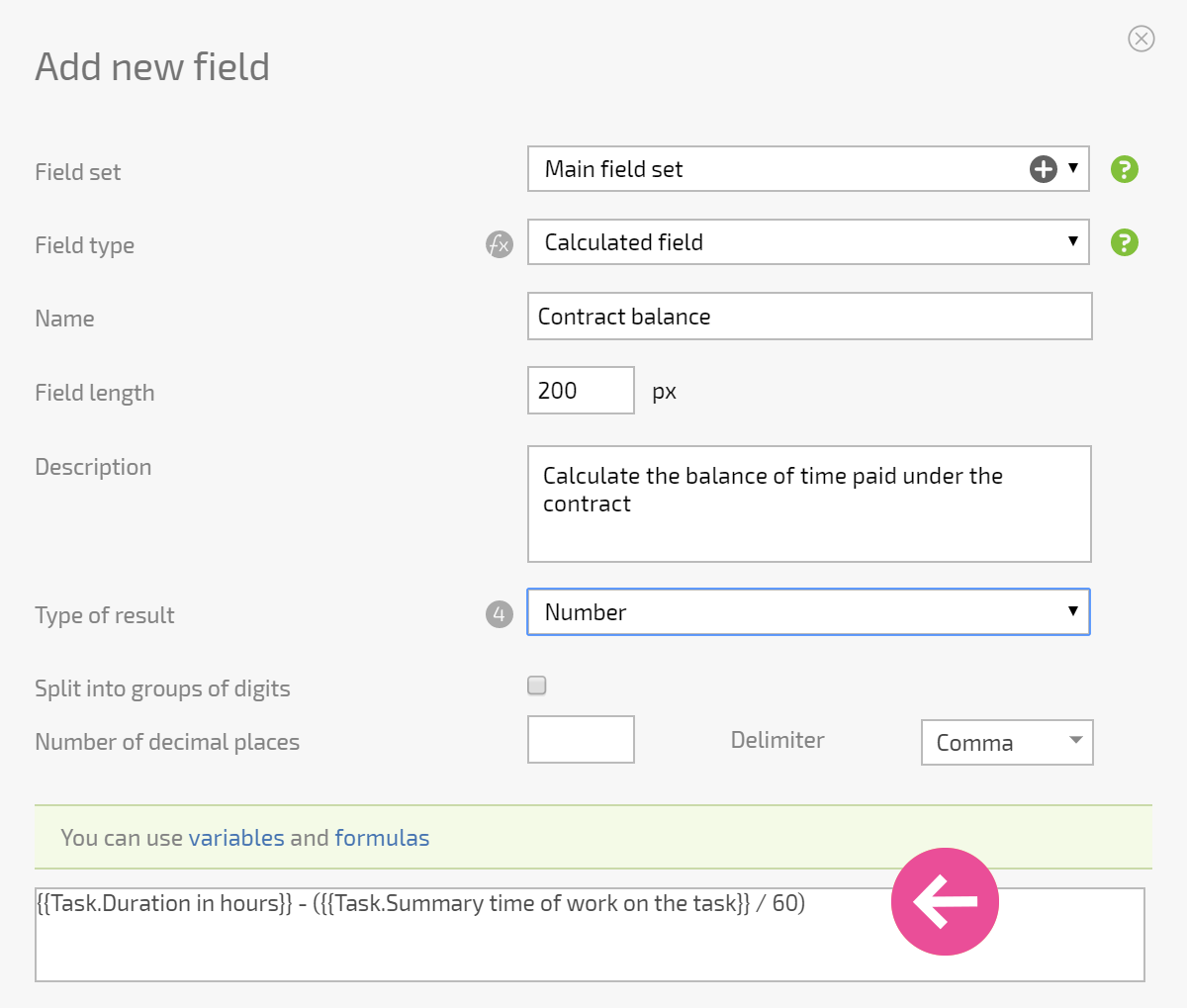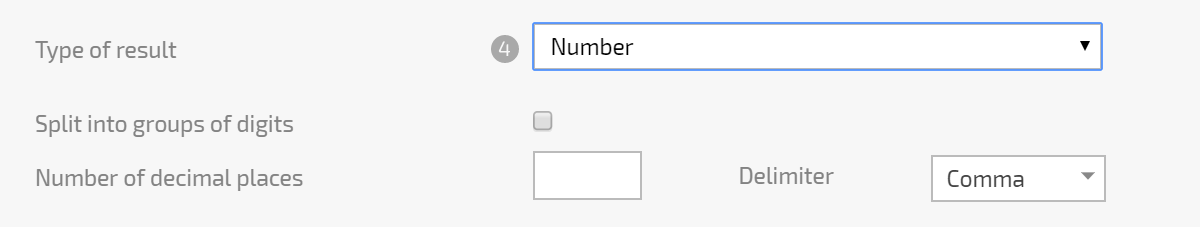A calculated field is a custom task field that lets you calculate a value you need, using data from various Planfix objects and standard Planfix functions.

## Calculated field settings

The key component of a calculated field is the formula used to calculate its value:You can also choose the format in which the value of your calculated field will be stored:## Important

• Calculated fields are calculated when a task is created or modified. If your formula uses a value from an object field referenced in a task (such as a field from a counterparty's task card) and this field changes, the calculated field value won't change. It will be recalculated only when a task parameter is changed that calls the Task modified event. You must keep this in mind when you use fields from embedded system objects.
• Calculated fields will not be calculated in tasks with the status Draft. Values will be calculated when the task is changed to have any of the working statuses.
• Calculated field formulas can contain values from other calculated fields.
• Your plan determines the maximum number of calculated fields you can add.